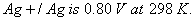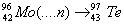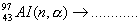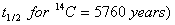Tuesday 29th September 2020

CBSE Guess > Papers > Question Papers > Class XII > 2003 > Chemistry > Compartment Delhi Set -II.

CHEMISTRY—2003 (Set II—Compartment Delhi)

Note: Except for the following questions, all the remaining questions have been asked in Set I.

Q. 1. What is the difference between glass and quartz, though both contain SiO 4 units? 1

Q. 7. What is the state of hybridization of nitrogen in the nitrate ion,1

Q. 8. Name two phenomena that indicate the dull nature of electrons. 1

Q. 10. On mixing equal volumes of water and ethanol, what type of deviation would you expect from Raoult's law? 1

Q. 11. Describe and explain what is observed when 2
(i) a beam of light is passed through a colloidal solution of As 2 S 3 .
(ii) an electric current Is passed through a colloidal solution.

Q. 14. Write the chemical equation representing the system of glyptal. Mention any one of its uses in industry. 2

Q. 26. The specific rate constant for a particular reaction is 2.34 x 10 -3 mol L -1 s -1 at 370 K and 7.50 x 10 -2 mol L -1 s -1 at 400 K. Calculate the activation energy for the reaction.
[R = 8.314 JK -1 mol -1]

Q. 28. (a) Using valence bond theory deduce the geometry and magnetic nature of [Ni (CN) 4 ] 2- .
(At. No. of Ni = 28)
(b) Name the geometrical isomers of [Co(en) 2CI 2 ]+ .
(en = ethylenediamine) 3

Q. 30. (a) Calculate the electrode potential of silver electrode dipped in a 0.1 M solution of silver nitrate at 298 K assuming silver nitrate to be completely dissociated. The standard electrode potential of(b) At what concentration of silver ions will this electrode have a potential of zero volt? 3

Q. 31. (a) Complete the following nuclear reactions:
(i)(ii)(b) A piece of wood from an old cave gives 6 counts per minute for one gram of carbon. How old is the piece of wood, if in a freshly cut wood the number of counts per minute per gram of carbon is 15.3? 3Chemistry 2003 Question Papers Class XII Delhi Outside Delhi Compartment Delhi Compartment Outside DelhiSet ISet ISet ISet ISet IISet IISet IISet II

CBSE 2003 Question Papers Class XII

>